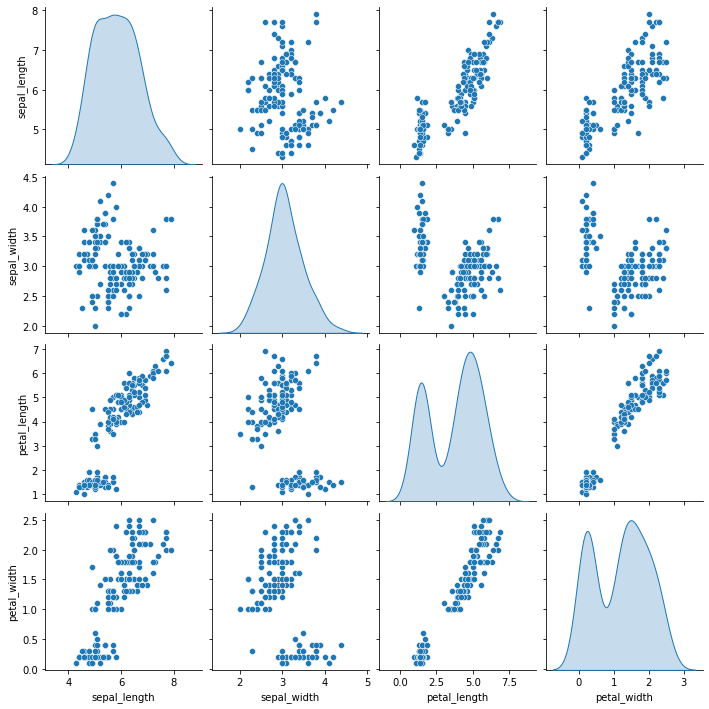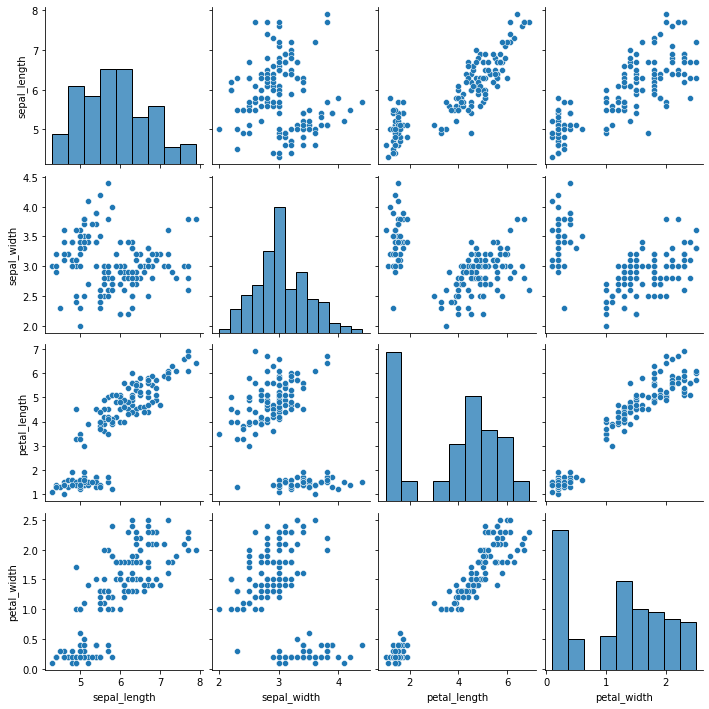# Custom Correlogram with Seaborn

This post shows how to custom the correlation matrix using seaborn pairplot() function with several reproducible code examples.

The previous post shows how to make a basic correlogram with seaborn. This post aims to explain how to improve it. It is divided in 2 parts: how to custom the correlation observation (for each pair of numeric variable), and how to custom the distribution (diagonal of the matrix).

## Correlation

The seaborn library allows to draw a correlation matrix through the `pairplot()` function. The parameters to create the example graphs are:

• `data` : dataframe
• `kind` : kind of plot to make (possible kinds are ‘scatter’, ‘kde’, ‘hist’, ‘reg’)
``````# library & dataset
import matplotlib.pyplot as plt
import seaborn as sns

# with regression
sns.pairplot(df, kind="reg")
plt.show()

# without regression
sns.pairplot(df, kind="scatter")
plt.show()``````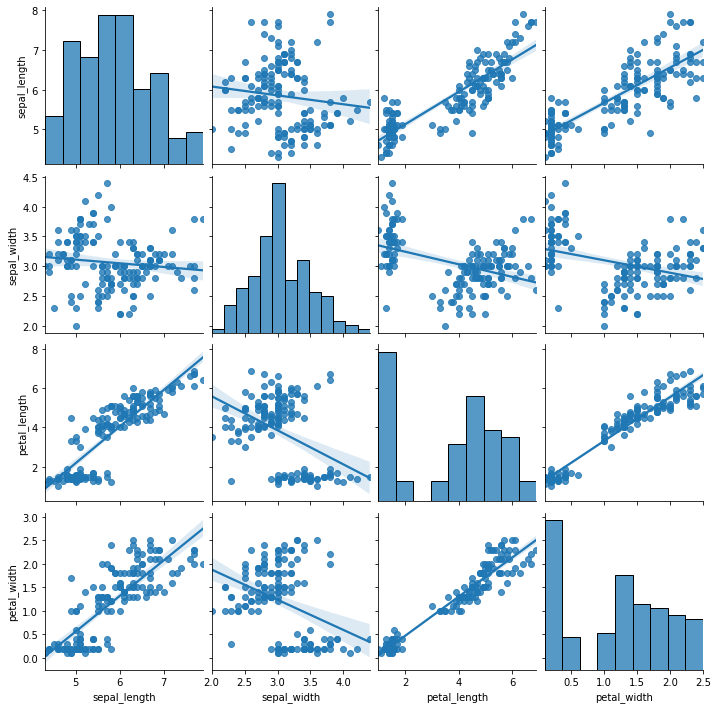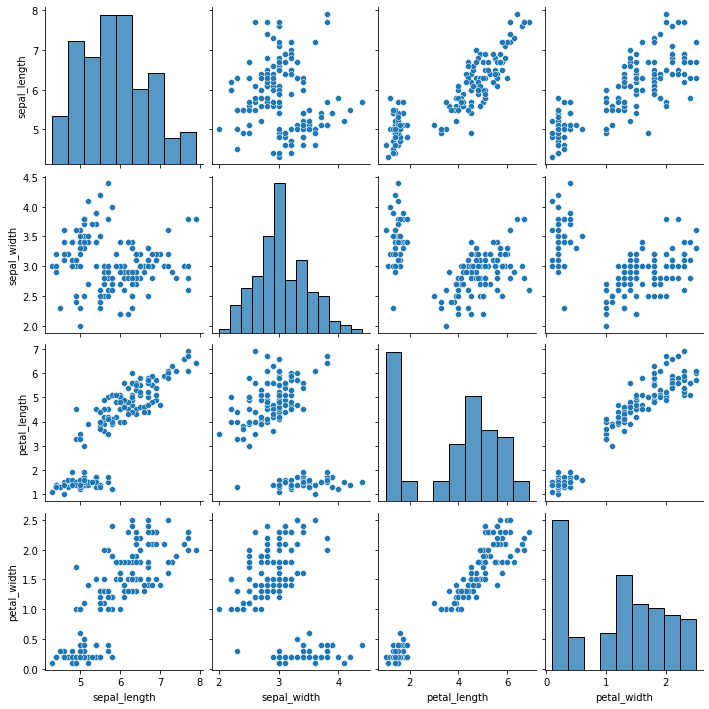In a graph drawn by `pairplot()` function of seaborn, you can control the marker features, colors and data groups by using additional parameters such as:

• `hue` : variables that define subsets of the data
• `markers` : a list of marker shapes
• `palette` : set of colors for mapping the hue variable
• `plot_kws` : a dictionary of keyword arguments to modificate the plot
``````# library & dataset
import matplotlib.pyplot as plt
import seaborn as sns

# left
sns.pairplot(df, kind="scatter", hue="species", markers=["o", "s", "D"], palette="Set2")
plt.show()

# right: you can give other arguments with plot_kws.
sns.pairplot(df, kind="scatter", hue="species", plot_kws=dict(s=80, edgecolor="white", linewidth=2.5))
plt.show()``````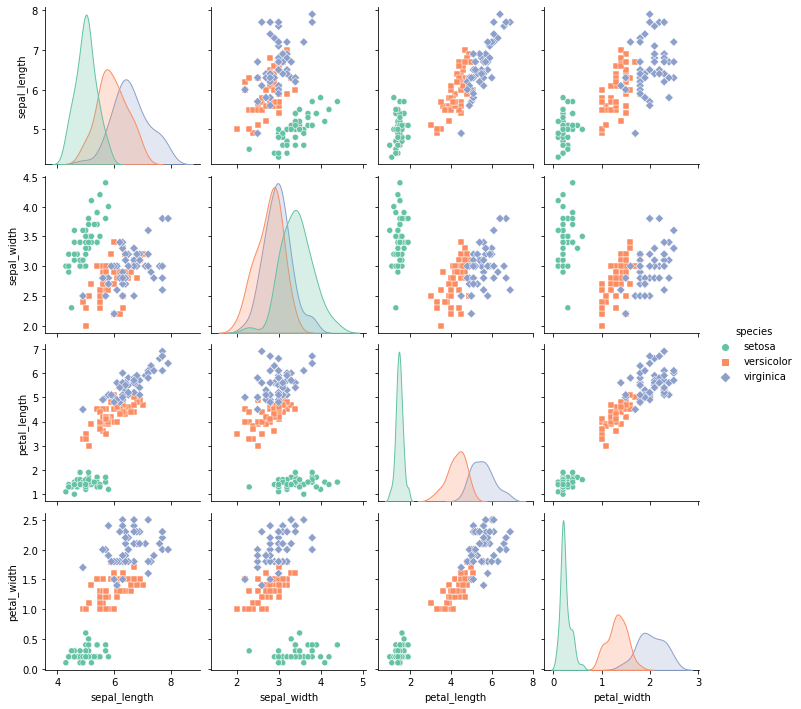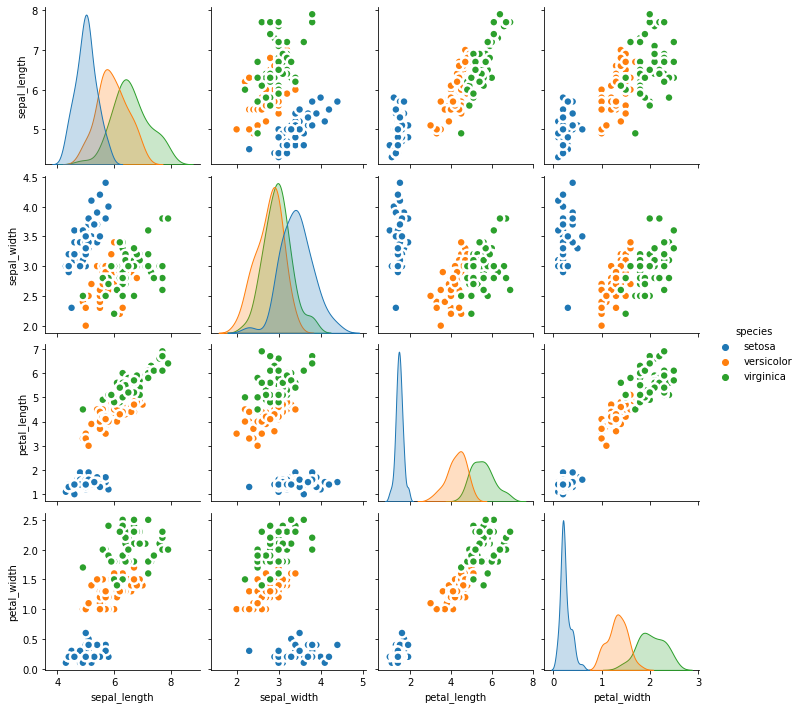## Distribution

As you can select the kind of plot to make in `pairplot()` function, you can also select the kind of plot for the diagonal subplots.

• `diag_kind` : the kind of plot for the diagonal subplots (possible kinds are ‘auto’, ‘hist’, ‘kde’, None)

Note that you can use `bw_adjust` to increase or decrease the amount of smoothing.

``````# library & dataset
import seaborn as sns
import matplotlib.pyplot as plt